# ICSE Selina Solution for Class 9 Chemistry Chapter 1 : The Language Of Chemistry

## ICSE Selina Concise Solution for Class 9 Chemistry Chapter 1- Free PDF Download

ICSE Selina Solution for Class 9 Chemistry Chapter 1 – The Language Of Chemistry provides you with detailed answers to all the exercise questions provided in Selina publication ICSE class 9 Chemistry textbook. This solution helps you to attain perfection in the topics involved in the chapter The Language Of Chemistry. To score good marks in Chemistry examination, students need to focus on this study material thoroughly.

This Class 9 Selina Solutions helps you to attain a basic knowledge of Chemistry such as symbols, valency, radicals, compounds, chemical equations, atomic weights, molecular weight and calculation of relative molecular mass and percentage composition of a compound.

ICSE Selina solutions act as a perfect study material that is required for your ICSE examination. Studying this ICSE Selina Solution for Class 9 Chemistry will also help you to get a basic knowledge of introduction to Chemistry.

Important topics covered in ICSE Selina Solution for Class 9 Chemistry Chapter 1 The Language Of Chemistry

• Introduction
• Symbols
• Valency
• Formula
• Writing chemical formulae
• Naming certain compounds
• Calculating the valency from the formula
• Chemical equation
• Relative atomic mass (Atomic weight)
• Relative molecular mass (Molecular weight)
• Percentage composition
• Empirical formula of a compound

### Download PDF of ICSE Selina Solution for Class 9 Chemistry Chapter 1 The Language Of Chemistry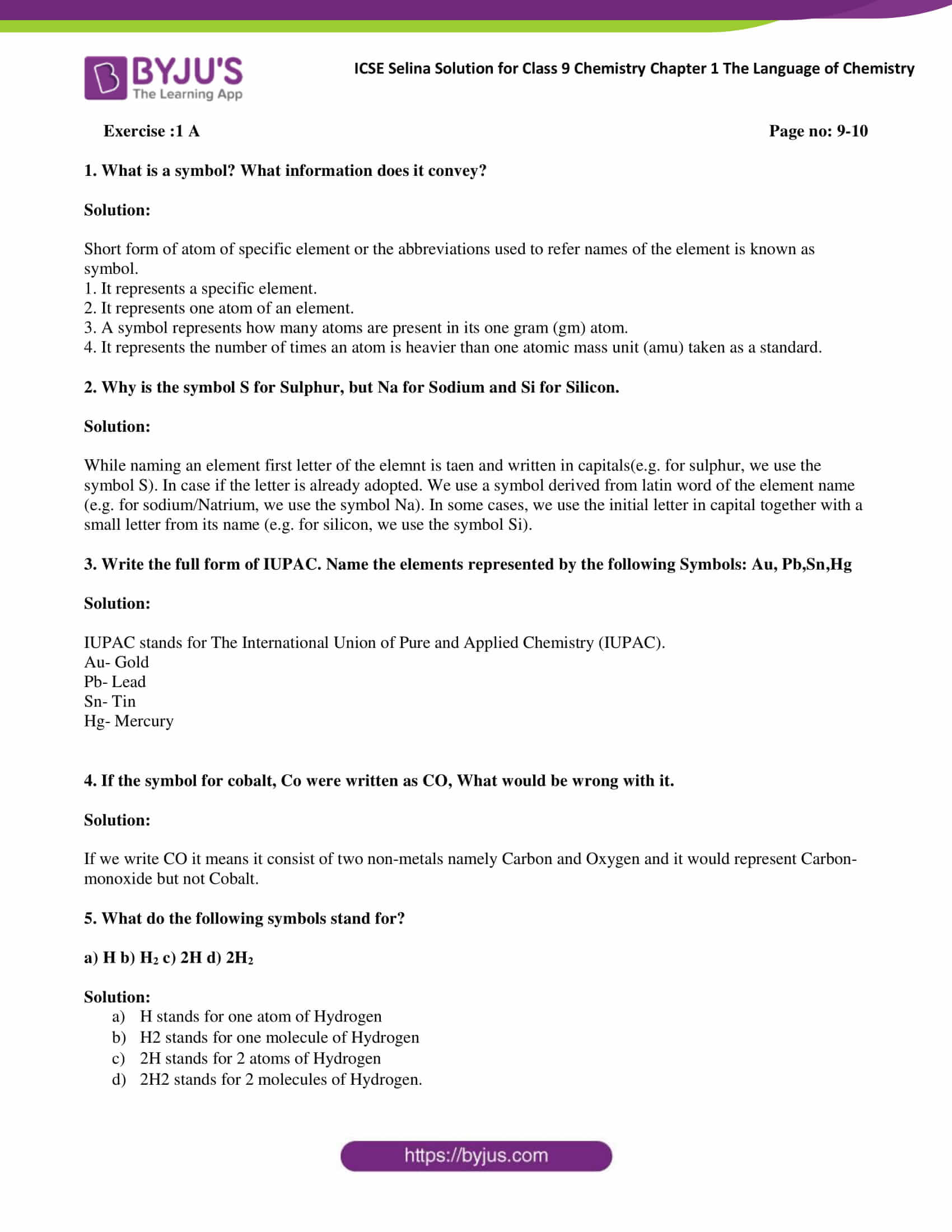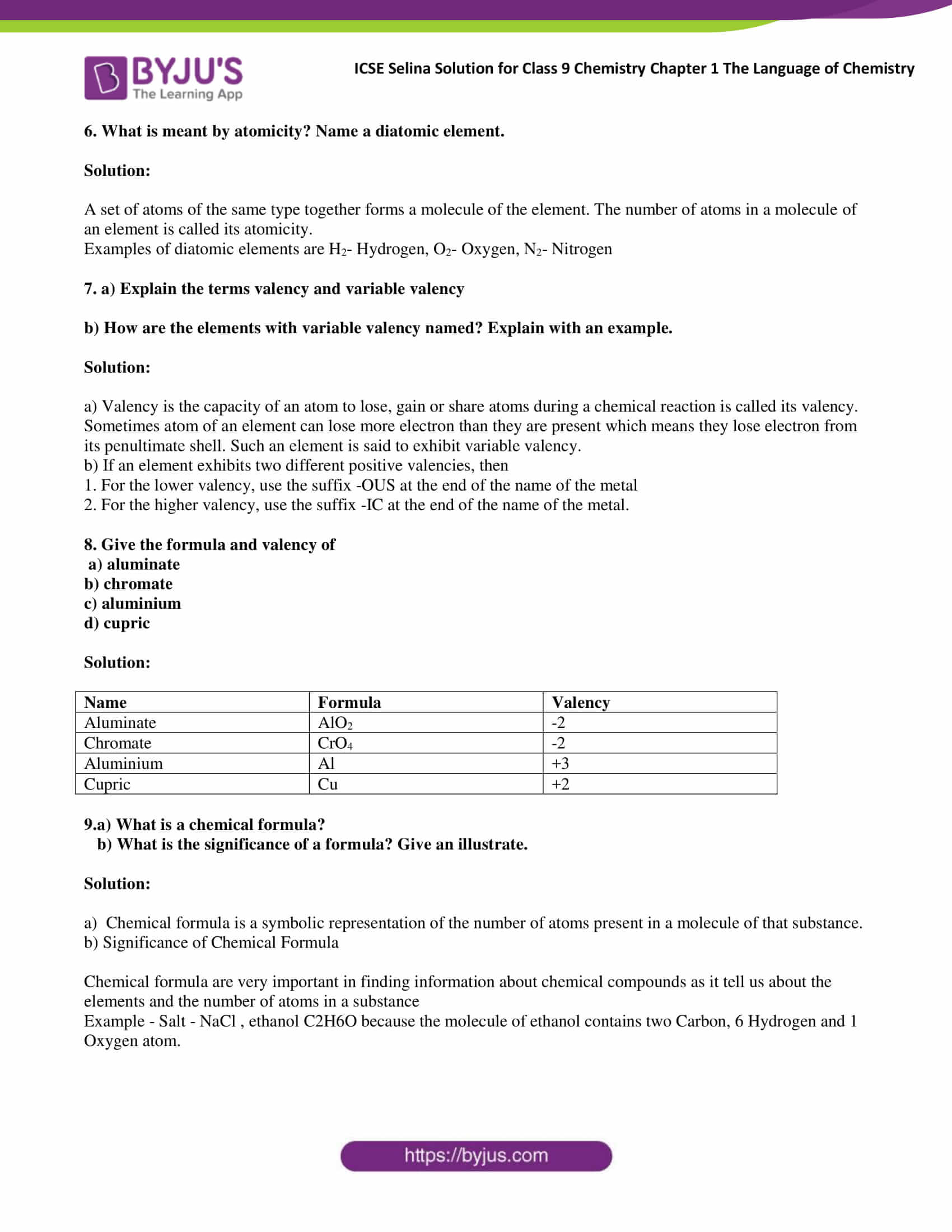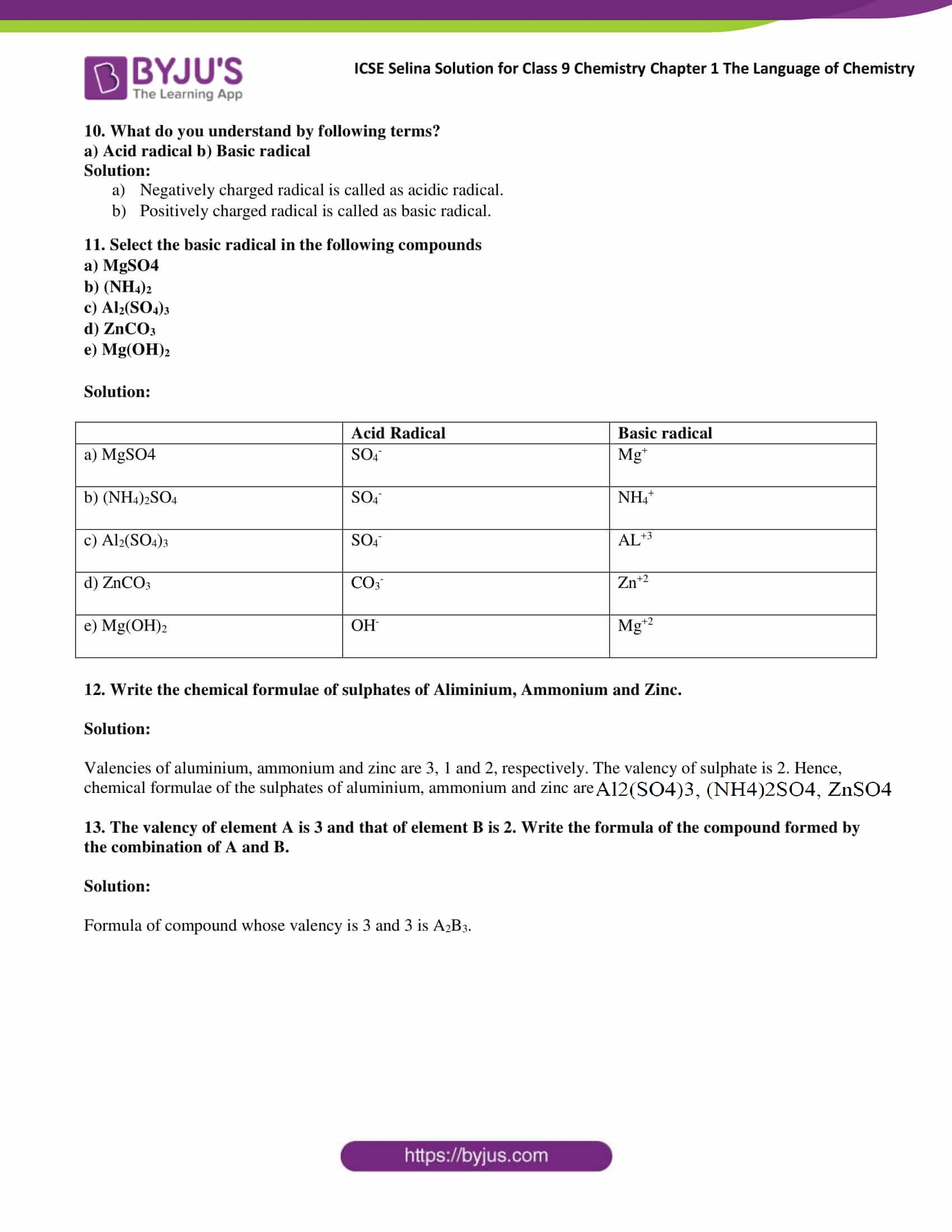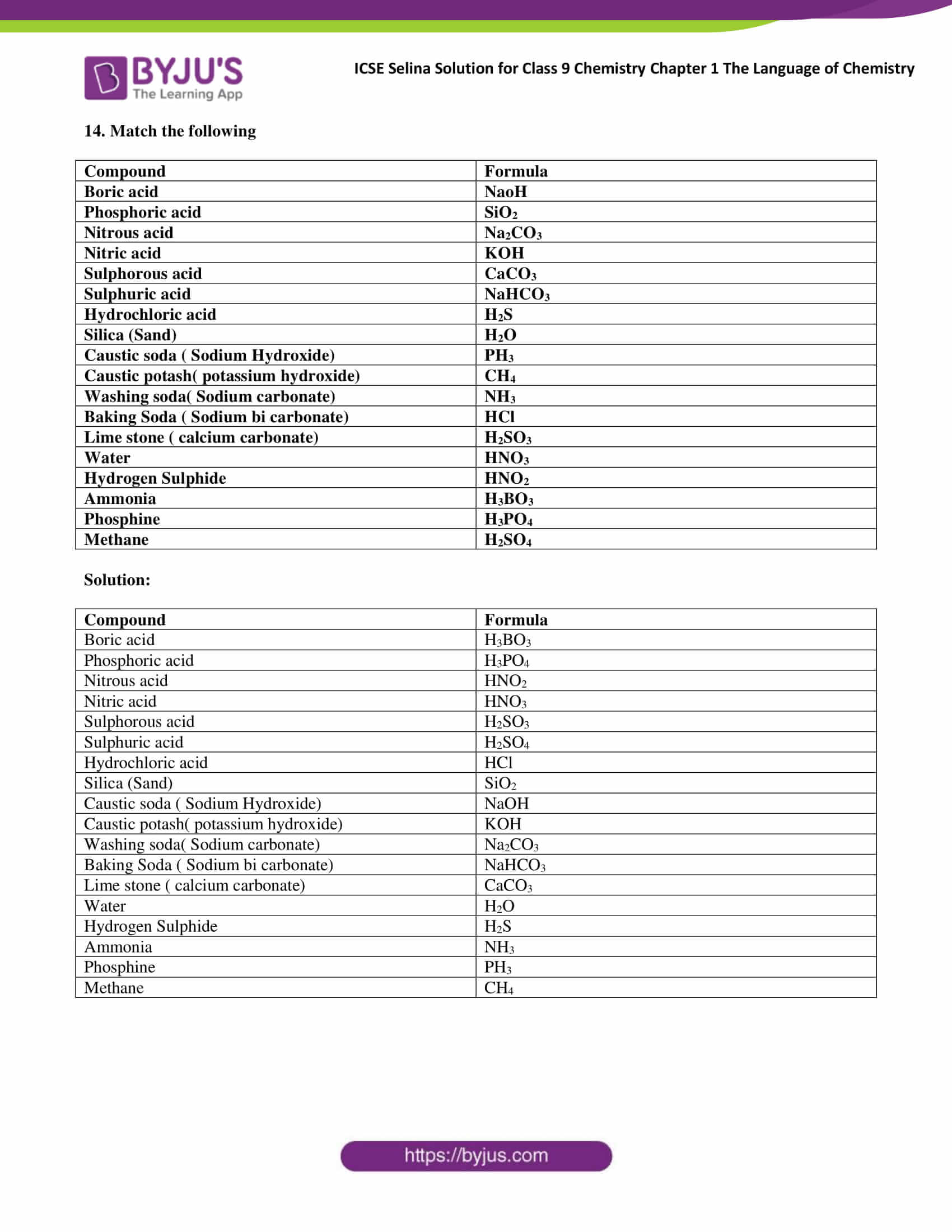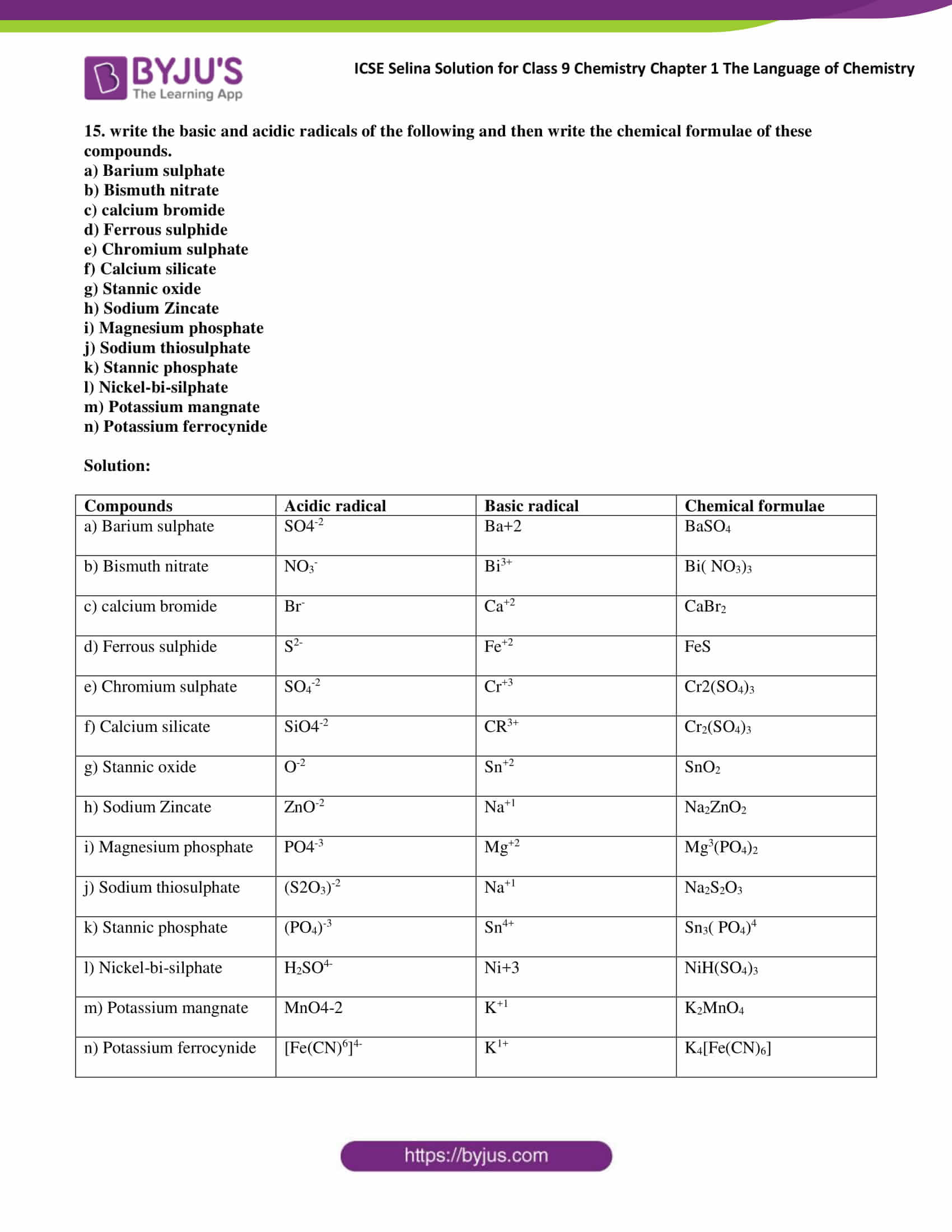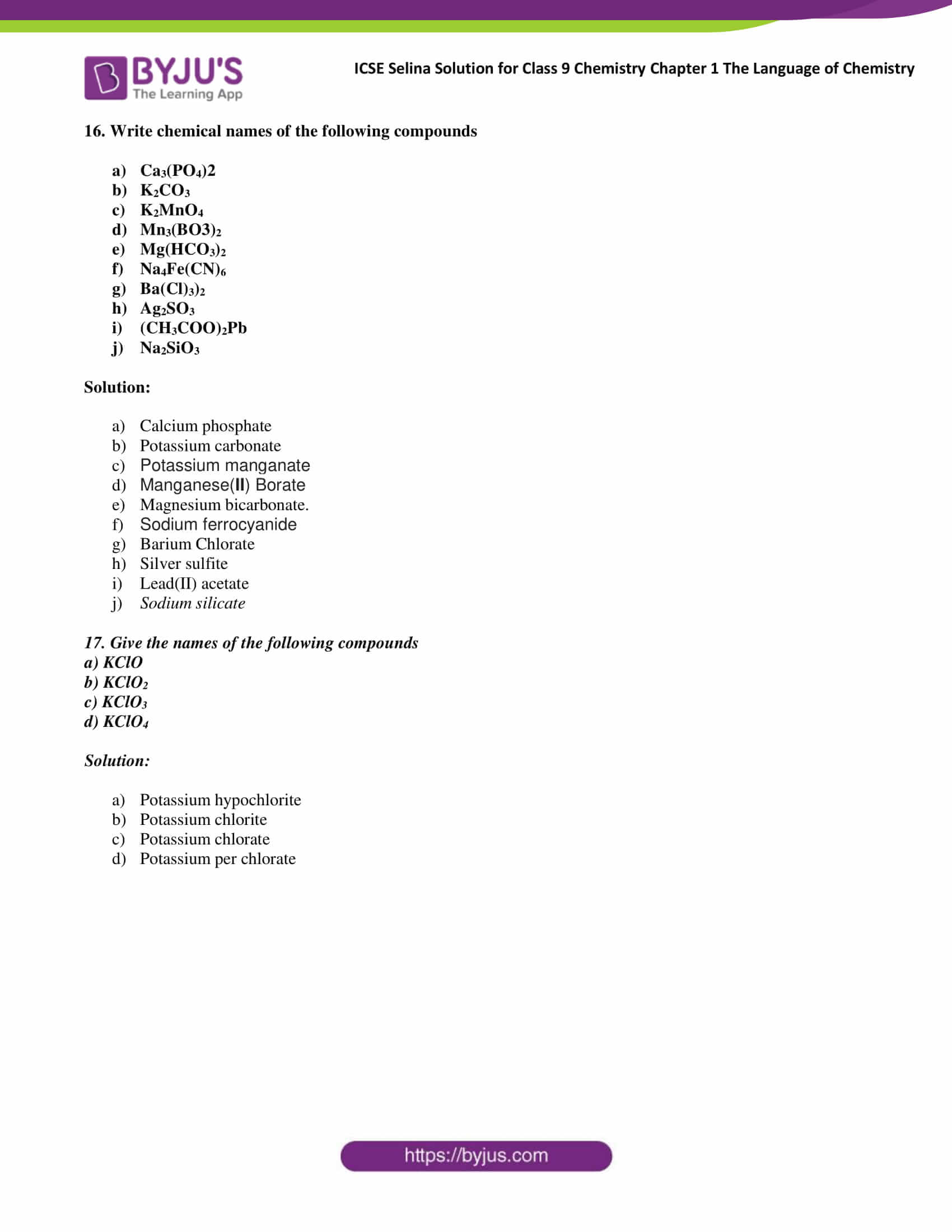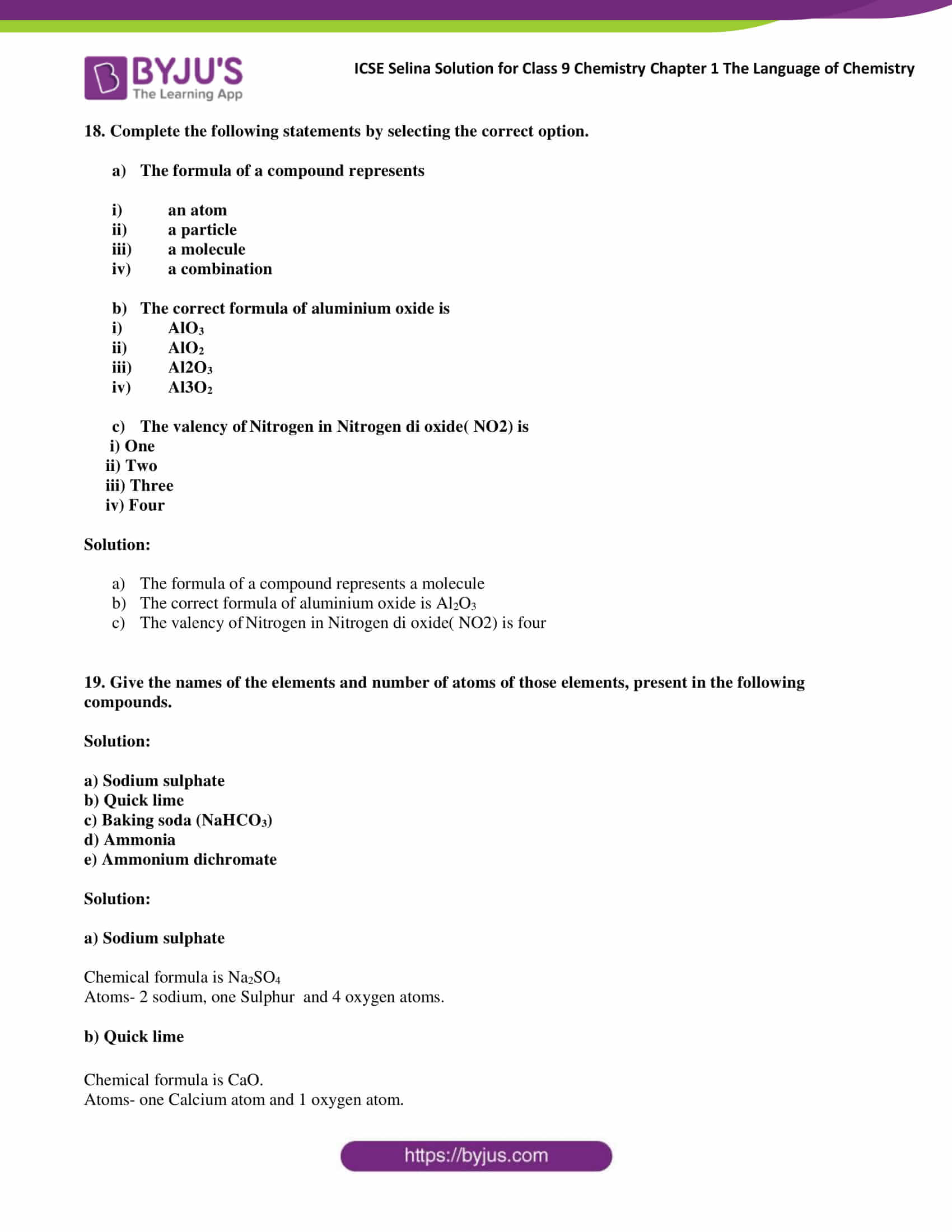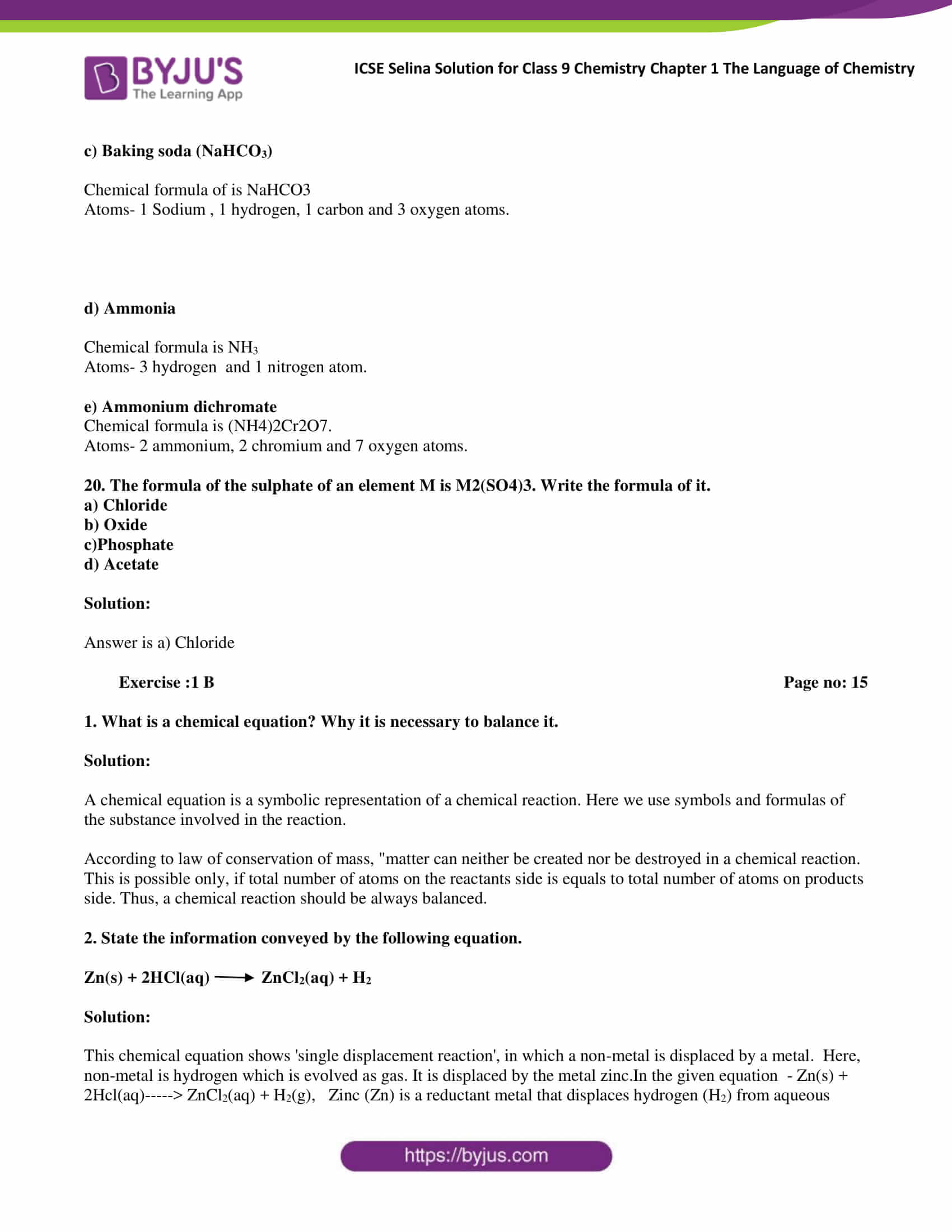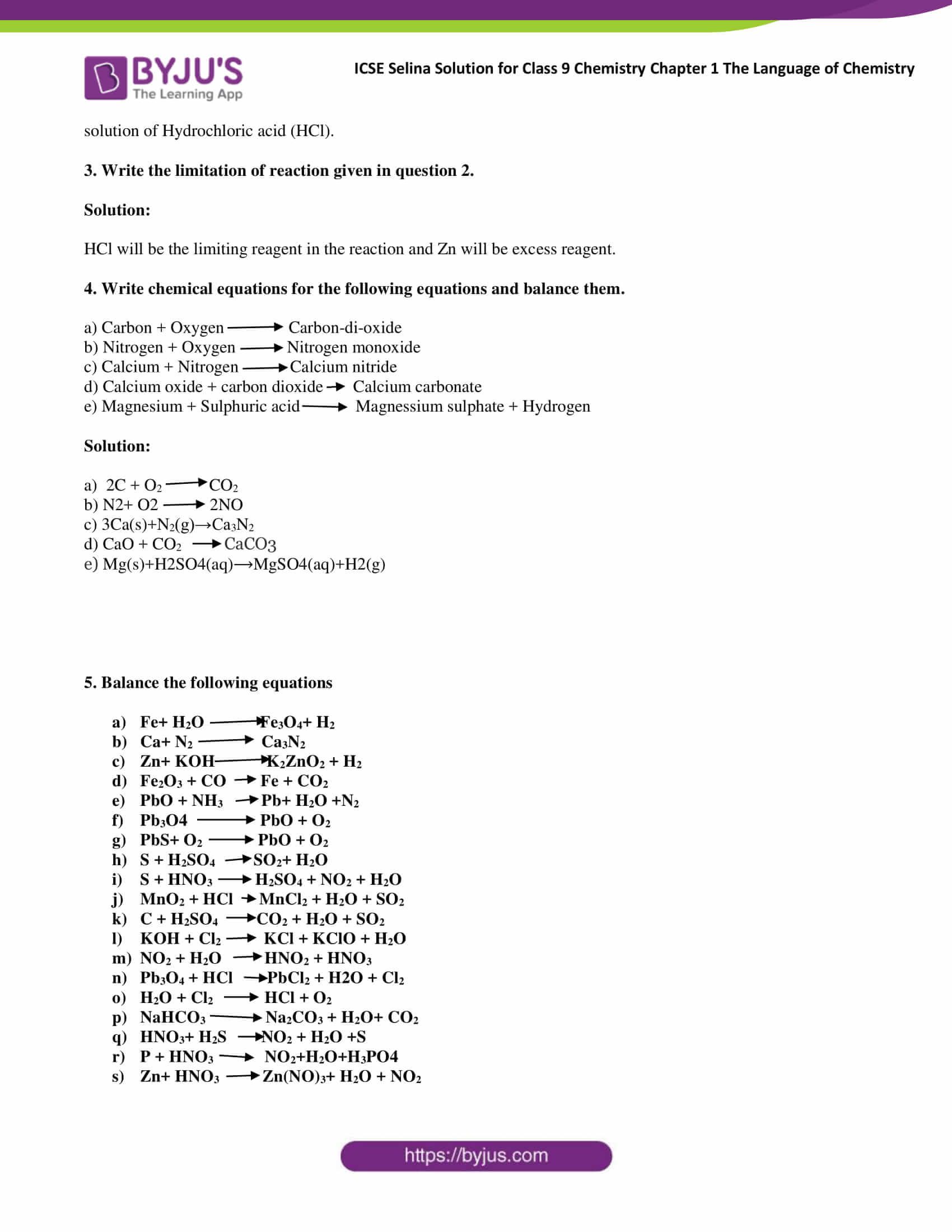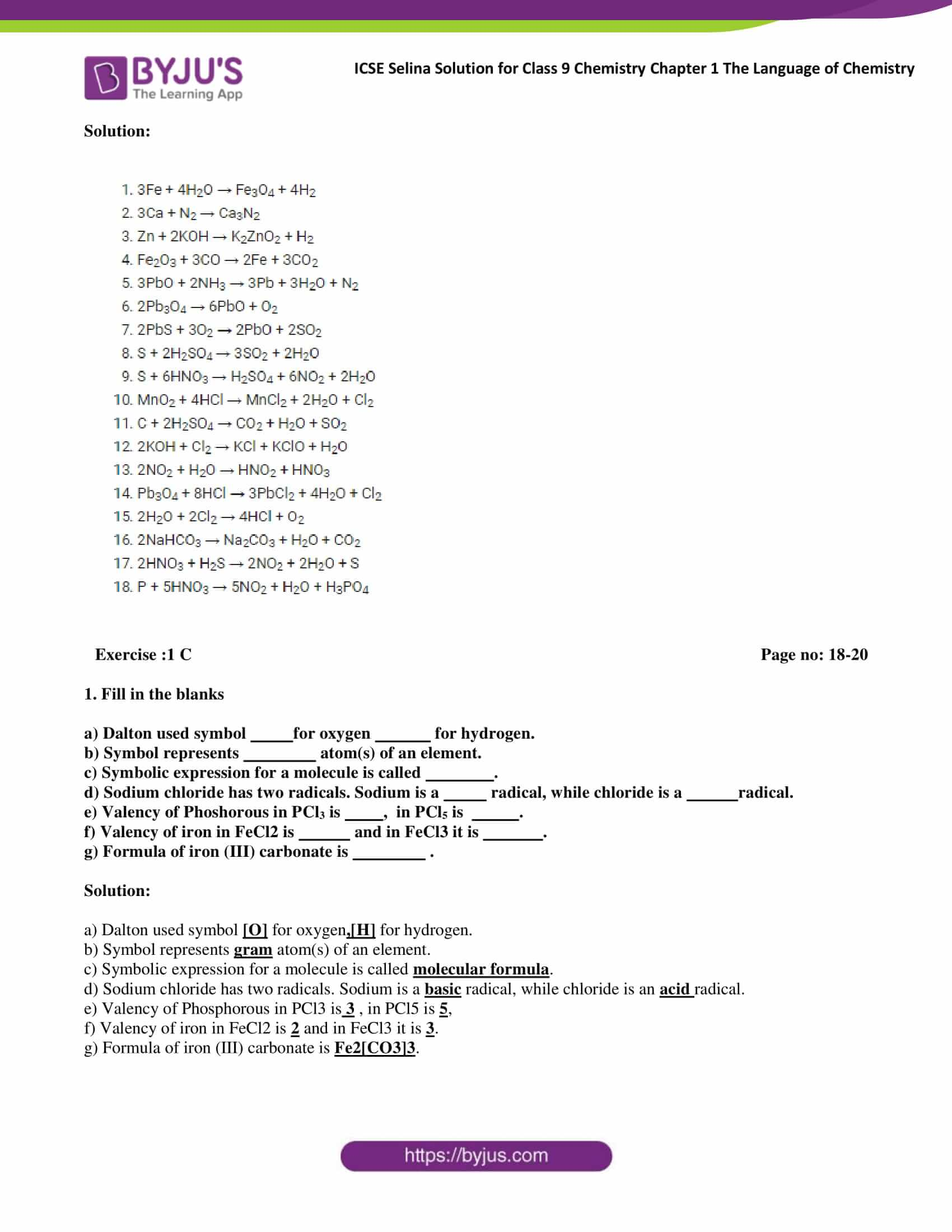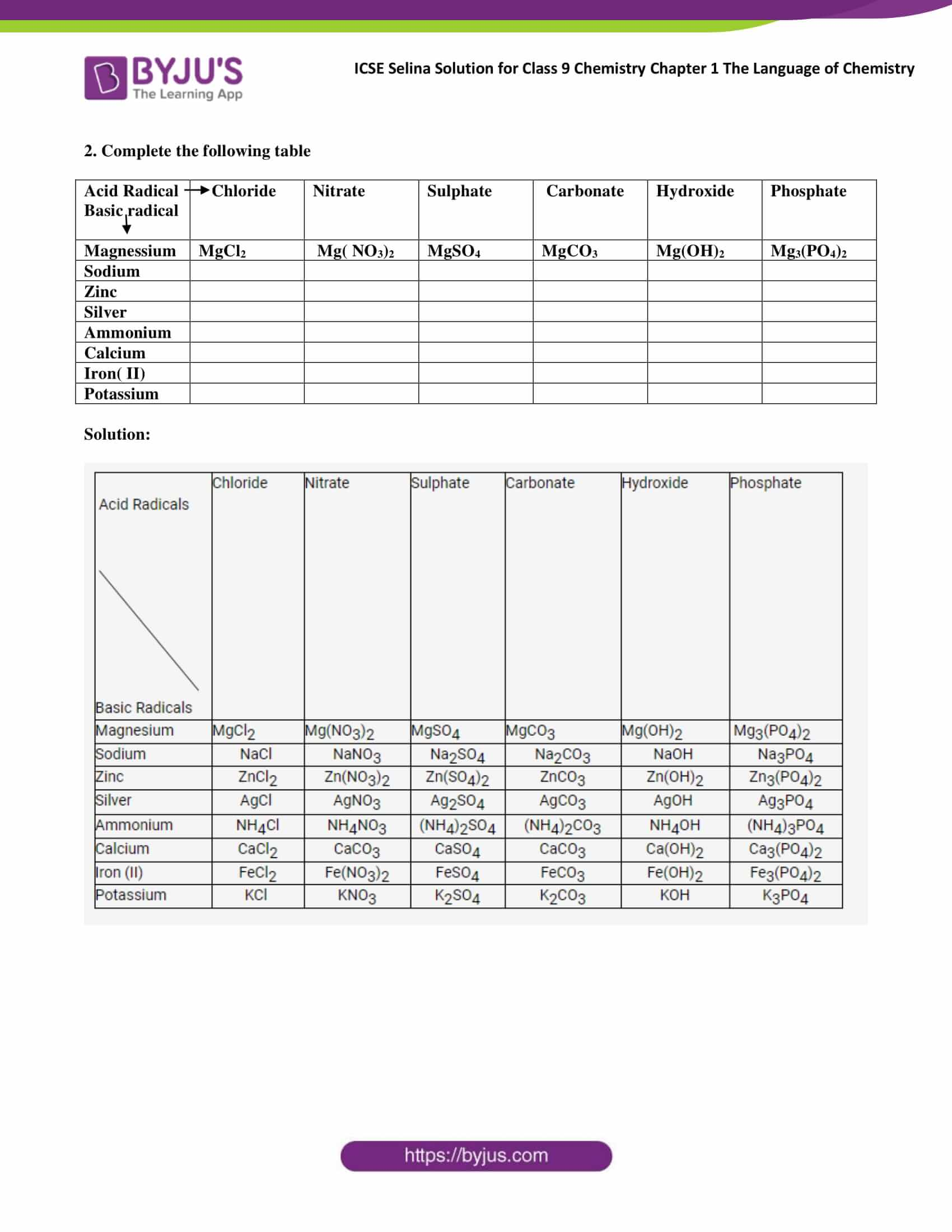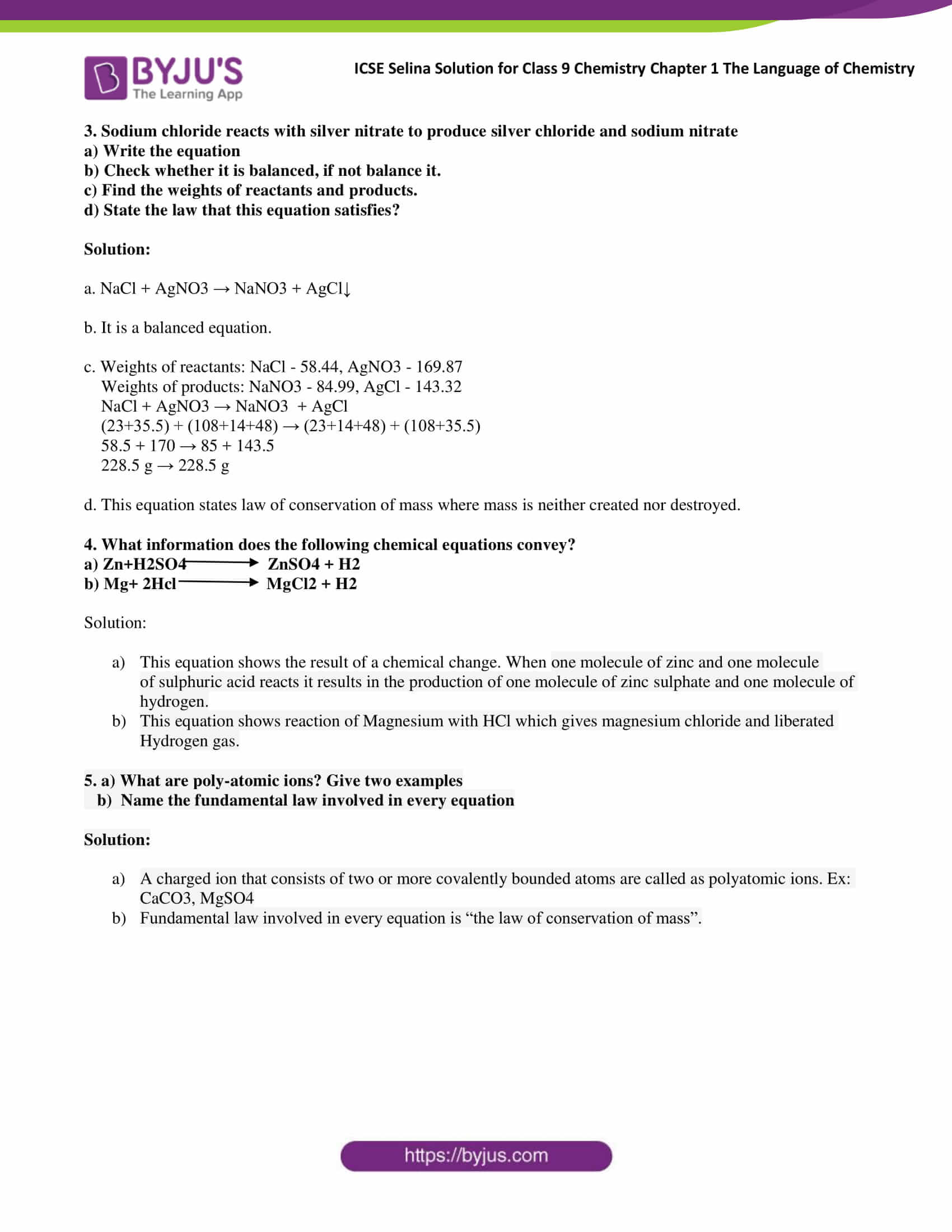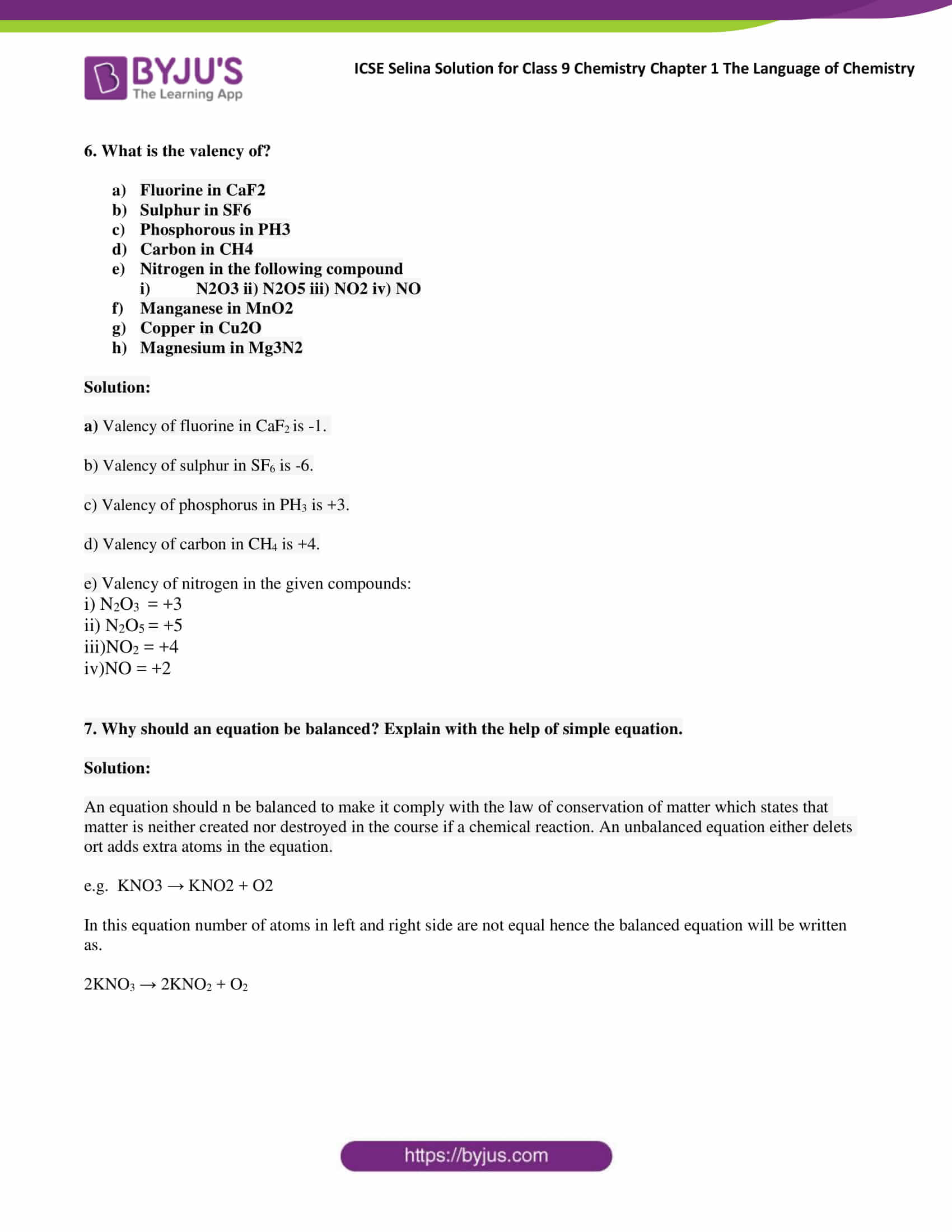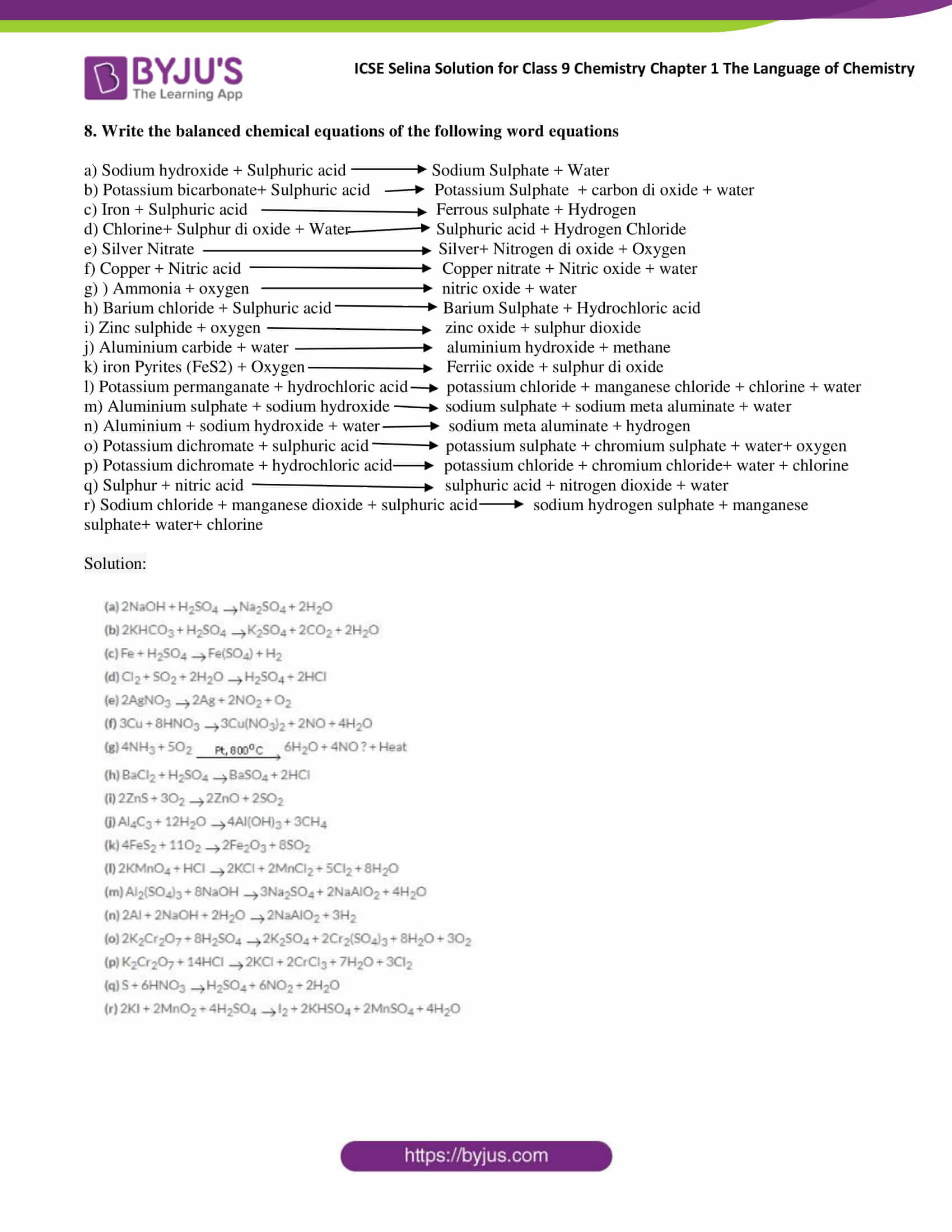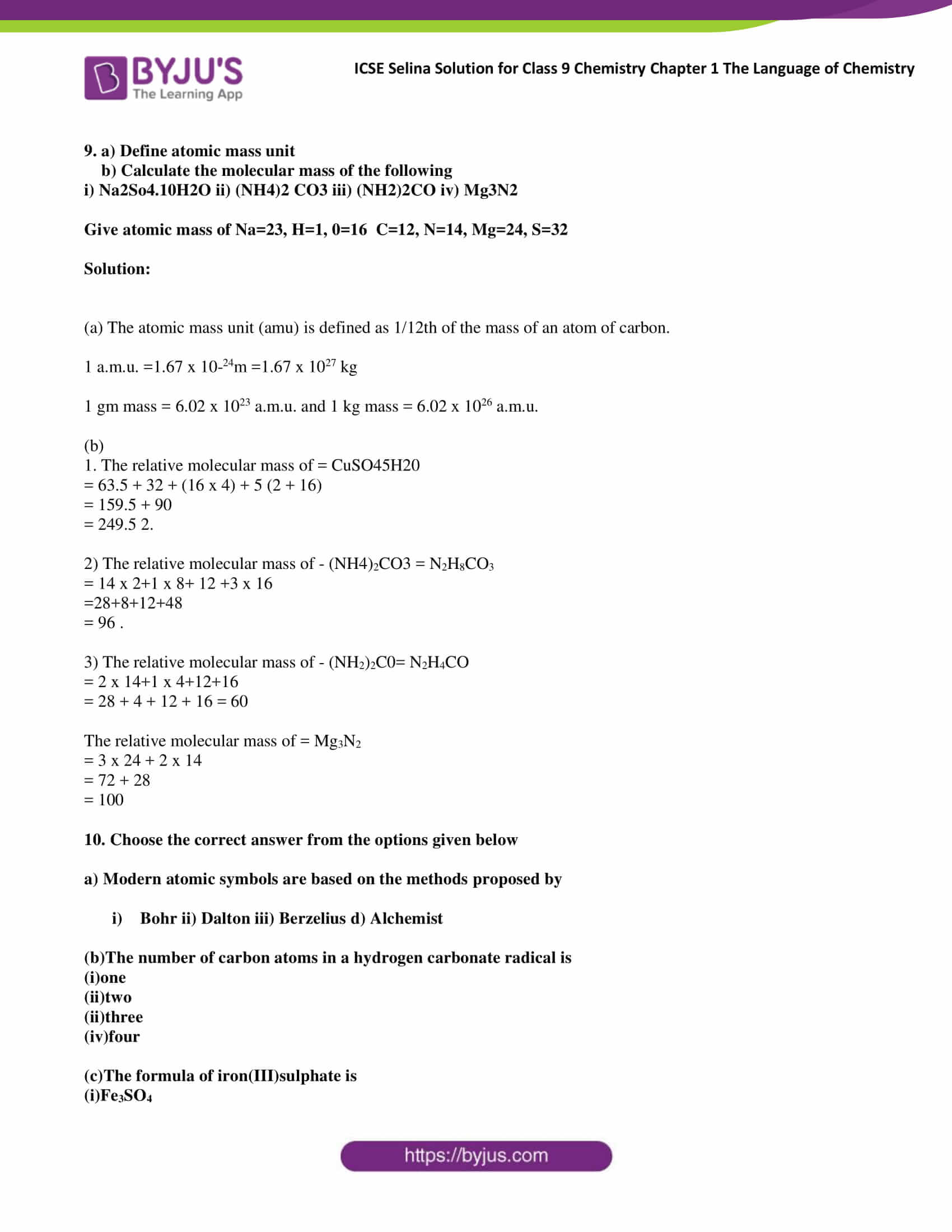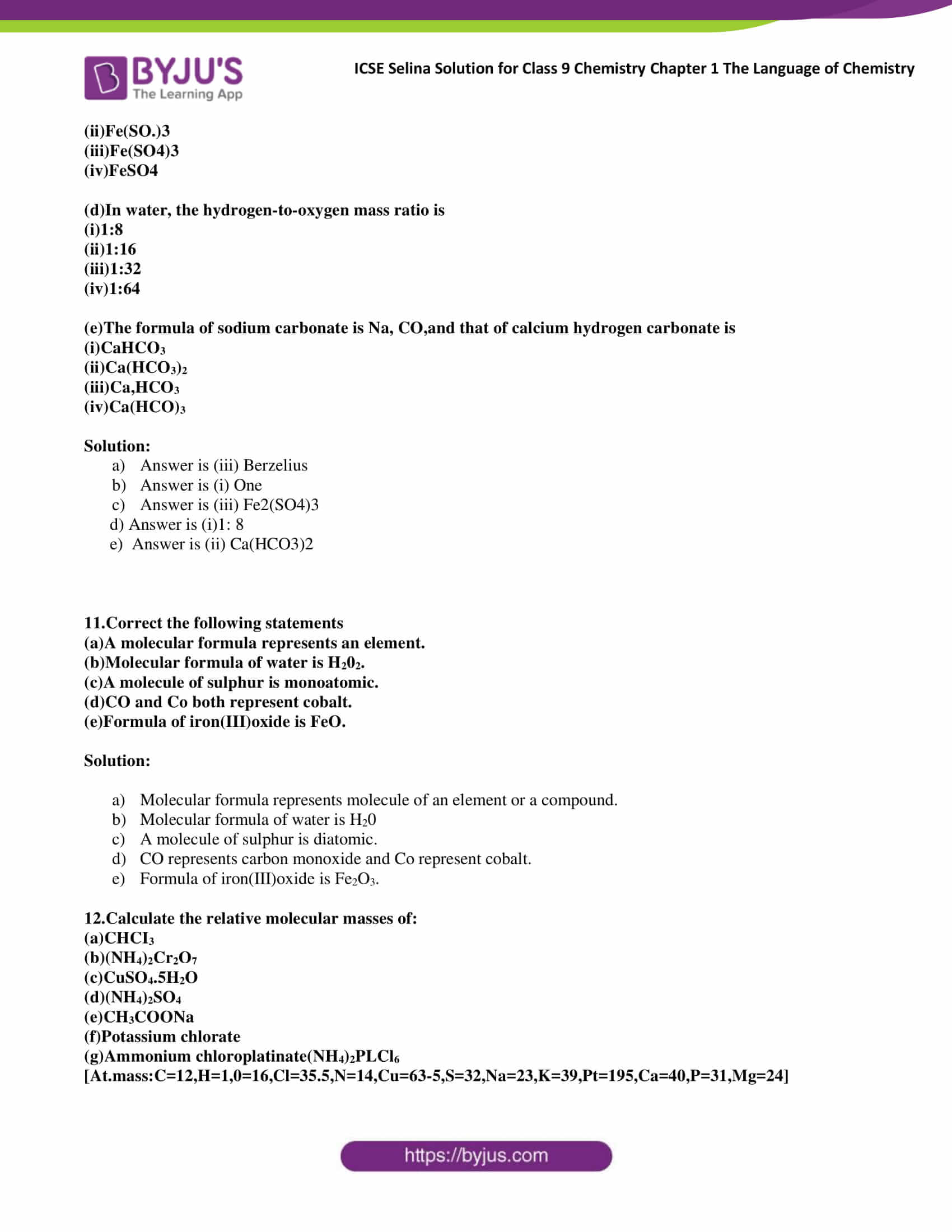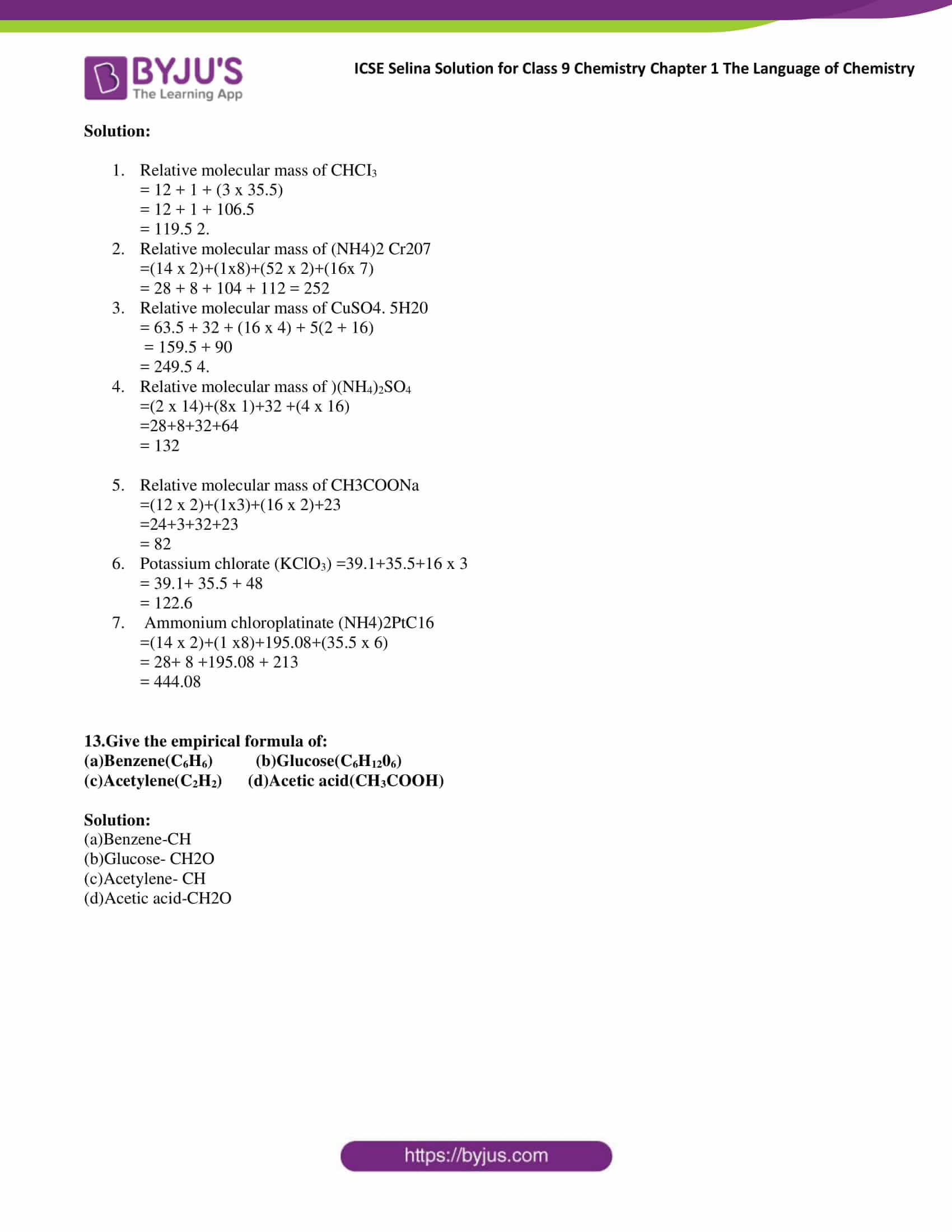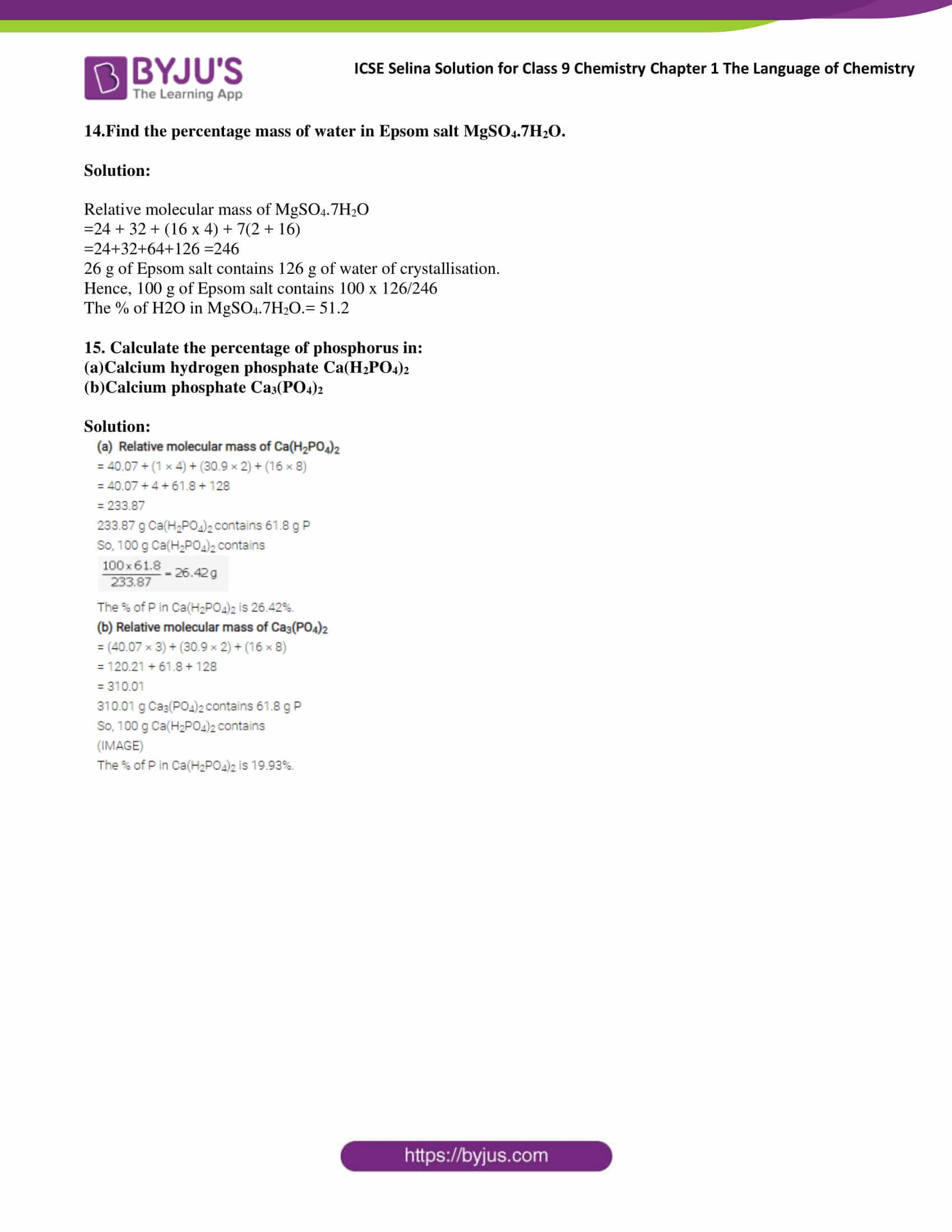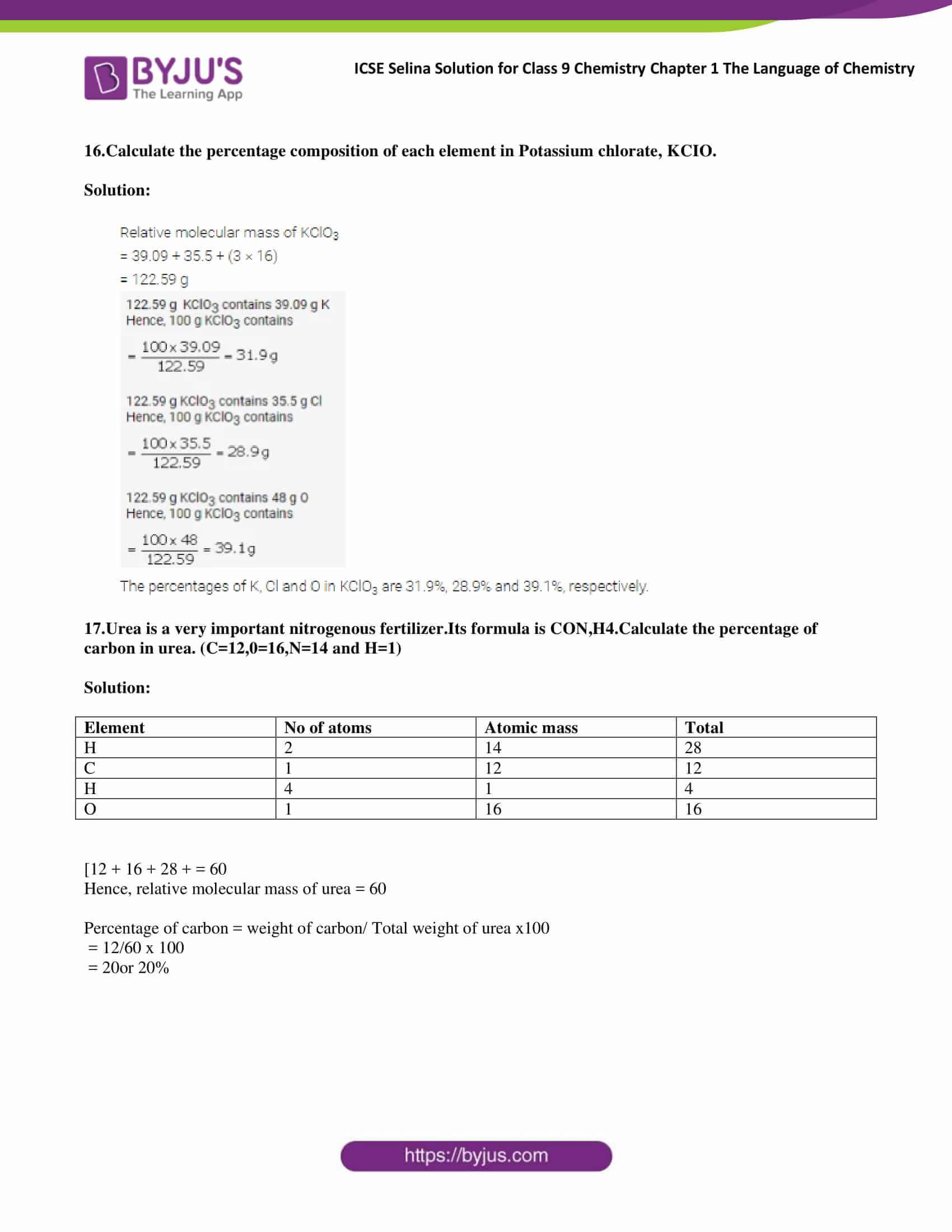### Access Answers of Selina publication ICSE Class 9 Chemistry Chapter 1 The Language Of Chemistry

Exercise:1

1. What is a symbol? What information does it convey?

Solution:

Short form of an atom of a specific element or the abbreviations used to refer names of the element is known as symbol.

1. It represents a specific element.

2. It represents one atom of an element.

3. A symbol represents how many atoms are present in its one gram (gm) atom.

4. It represents the number of times an atom is heavier than one atomic mass unit (amu) taken as a standard.

2. Why is the symbol S for Sulphur, but Na for Sodium and Si for Silicon?

Solution:

While naming an element first letter of the element is taken and written in capitals(e.g. for sulphur, we use the symbol S). In case if the letter is already adopted. We use a symbol derived from the Latin word of the element name (e.g. for sodium/Natrium, we use the symbol Na). In some cases, we use the initial letter in capital together with a small letter from its name (e.g. for silicon, we use the symbol Si).

3. Write the full form of IUPAC. Name the elements represented by the following Symbols: Au, Pb, Sn, Hg

Solution:

IUPAC stands for The International Union of Pure and Applied Chemistry (IUPAC).

Au- Gold

Sn- Tin

Hg- Mercury

4. If the symbol for cobalt, Co were written as CO, What would be wrong with it?

Solution:

If we write CO it means it consists of two non-metals namely Carbon and Oxygen and it would represent Carbon-monoxide but not Cobalt.

5. What do the following symbols stand for?

a) H b) H2 c) 2H d) 2H2

Solution:

1. H stands for one atom of Hydrogen
2. H2 stands for one molecule of Hydrogen
3. 2H stands for 2 atoms of Hydrogen
4. 2H2 stands for 2 molecules of Hydrogen.

6. What is meant by atomicity? Name a diatomic element.

Solution:

A set of atoms of the same type together forms a molecule of the element. The number of atoms in a molecule of an element is called its atomicity.

Examples of diatomic elements are H2– Hydrogen, O2– Oxygen, N2– Nitrogen

7. a) Explain the terms valency and variable valency

b) How are the elements with variable valency named? Explain with an example.

Solution:

a) Valency is the capacity of an atom to lose, gain or share atoms during a chemical reaction is called its valency. Sometimes atom of an element can lose more electron than they are present which means they lose electron from its penultimate shell. Such an element is said to exhibit variable valency.

b) If an element exhibits two different positive valencies, then

1. For the lower valency, use the suffix -OUS at the end of the name of the metal

2. For the higher valency, use the suffix -IC at the end of the name of the metal.

8. Give the formula and valency of

a) aluminate

b) chromate

c) aluminium

d) cupric

Solution:

 Name Formula Valency Aluminate AlO2 -2 Chromate CrO4 -2 Aluminium Al +3 Cupric Cu +2

9.a) What is a chemical formula?

b) What is the significance of a formula? Give an illustrate.

Solution:

a) A chemical formula is a symbolic representation of the number of atoms present in a molecule of that substance.

b) Significance of Chemical Formula

A chemical formula is very important in finding information about chemical compounds as it tells us about the elements and the number of atoms in a substance

Example – Salt – NaCl, ethanol C2H6O because the molecule of ethanol contains two Carbon, 6 Hydrogen and 1 Oxygen atom.

10. What do you understand by the following terms?

Solution:

11. Select the basic radical in the following compounds

a) MgSO4

b) (NH4)2

c) Al2(SO4)3

d) ZnCO3

e) Mg(OH)2

Solution:

 Acid Radical Basic radical a) MgSO4 SO4– Mg+ b) (NH4)2SO4 SO4– NH4+ c) Al2(SO4)3 SO4– Al+3 d) ZnCO3 CO3– Zn+2 e) Mg(OH)2 OH– Mg+2

12. Write the chemical formulae of sulphates of Aluminium, Ammonium and Zinc.

Solution:

Valencies of aluminium, ammonium and zinc are 3, 1 and 2, respectively. The valency of sulphate is 2. Hence, chemical formulae of the sulphates of aluminium, ammonium and zinc are Al2(SO4)3, (NH4)2SO4, ZnSO4.

13. The valency of element A is 3 and that of element B is 2. Write the formula of the compound formed by the combination of A and B.

Solution:

The formula of the compound whose valency is 3 and 3 is A2B3.

14. Match the following

 Compound Formula Boric acid NaOH Phosphoric acid SiO2 Nitrous acid Na2CO3 Nitric acid KOH Sulphorous acid CaCO3 Sulphuric acid NaHCO3 Hydrochloric acid H2S Silica (Sand) H2O Caustic soda ( Sodium Hydroxide) PH3 Caustic potash( potassium hydroxide) CH4 Washing soda( Sodium carbonate) NH3 Baking Soda ( Sodium bicarbonate) HCl Limestone ( calcium carbonate) H2SO3 Water HNO3 Hydrogen Sulphide HNO2 Ammonia H3BO3 Phosphine H3PO4 Methane H2SO4

Solution:

 Compound Formula Boric acid H3BO3 Phosphoric acid H3PO4 Nitrous acid HNO2 Nitric acid HNO3 Sulphorous acid H2SO3 Sulphuric acid H2SO4 Hydrochloric acid HCl Silica (Sand) SiO2 Caustic soda ( Sodium Hydroxide) NaOH Caustic potash( potassium hydroxide) KOH Washing soda( Sodium carbonate) Na2CO3 Baking Soda ( Sodium bicarbonate) NaHCO3 Limestone ( calcium carbonate) CaCO3 Water H2O Hydrogen Sulphide H2S Ammonia NH3 Phosphine PH3 Methane CH4

15. write the basic and acidic radicals of the following and then write the chemical formulae of these compounds.

a) Barium sulphate

b) Bismuth nitrate

c) calcium bromide

d) Ferrous sulphide

e) Chromium sulphate

f) Calcium silicate

g) Stannic oxide

h) Sodium Zincate

i) Magnesium phosphate

j) Sodium thiosulphate

k) Stannic phosphate

l) Nickel-bi-sulphate

m) Potassium mangnate

n) Potassium ferrocynide

Solution:

 Compounds Acidic radical Basic radical Chemical formulae a) Barium sulphate SO4-2 Ba+2 BaSO4 b) Bismuth nitrate NO3– Bi3+ Bi( NO3)3 c) calcium bromide Br– Ca+2 CaBr2 d) Ferrous sulphide S2- Fe+2 FeS e) Chromium sulphate SO4-2 Cr+3 Cr2(SO4)3 f) Calcium silicate SiO4-2 CR3+ Cr2(SO4)3 g) Stannic oxide O-2 Sn+2 SnO2 h) Sodium Zincate ZnO-2 Na+1 Na2ZnO2 i) Magnesium phosphate PO4-3 Mg+2 Mg3(PO4)2 j) Sodium thiosulphate (S2O3)-2 Na+1 Na2S2O3 k) Stannic phosphate (PO4)-3 Sn4+ Sn3( PO4)4 l) Nickel-bi-silphate H2SO4- Ni+3 NiH(SO4)3 m) Potassium mangnate MnO4-2 K+1 K2MnO4 n) Potassium ferrocynide [Fe(CN)6]4- K1+ K4[Fe(CN)6]

16. Write chemical names of the following compounds

1. Ca3(PO4)2
2. K2CO3
3. K2MnO4
4. Mn3(BO3)2
5. Mg(HCO3)2
6. Na4Fe(CN)6
7. Ba(Cl)3)2
8. Ag2SO3
9. (CH3COO)2Pb
10. Na2SiO3

Solution:

1. Calcium phosphate
2. Potassium carbonate
3. Potassium manganate
4. Manganese(II) Borate
5. Magnesium bicarbonate.
6. Sodium ferrocyanide
7. Barium Chlorate
8. Silver sulfite
10. Sodium silicate

17. Give the names of the following compounds

a) KClO

b) KClO2

c) KClO3

d) KClO4

Solution:

1. Potassium hypochlorite
2. Potassium chlorite
3. Potassium chlorate
4. Potassium perchlorate

18. Complete the following statements by selecting the correct option.

a)The formula of a compound represents

1. an atom
2. a particle
3. a molecule
4. a combination

b) The correct formula of aluminium oxide is

1. AlO3
2. AlO2
3. Al2O3
4. Al3O2

c) The valency of Nitrogen in Nitrogen dioxide( NO2) is

i) One

ii) Two

iii) Three

iv) Four

Solution:

1. The formula of a compound represents a molecule
2. The correct formula of aluminium oxide is Al2O3
3. The valency of Nitrogen in Nitrogen dioxide( NO2) is four

19. Give the names of the elements and number of atoms of those elements, present in the following compounds.

Solution:

a) Sodium sulphate

b) Quick lime

c) Baking soda (NaHCO3)

d) Ammonia

e) Ammonium dichromate

Solution:

a) Sodium sulphate

A chemical formula is Na2SO4

Atoms- 2 sodium, one Sulphur and 4 oxygen atoms.

b) Quick lime

The chemical formula is CaO.

Atoms- one Calcium atom and 1 oxygen atom.

c) Baking soda (NaHCO3)

The chemical formula is NaHCO3

Atoms- 1 Sodium, 1 hydrogen, 1 carbon and 3 oxygen atoms.

d) Ammonia

A chemical formula is NH3

Atoms- 3 hydrogens and 1 nitrogen atom.

e) Ammonium dichromate

The chemical formula is (NH4)2Cr2O7.

Atoms- 2 ammonium, 2 chromium and 7 oxygen atoms.

20. The formula of the sulphate of an element M is M2(SO4)3. Write the formula of it.

a) Chloride

b) Oxide

c)Phosphate

d) Acetate

Solution:

## Frequently Asked Questions on The Language of Chemistry

### What is a symbol?

Short form of an atom of a specific element or the abbreviations used to refer names of the element is known as symbol.

### Full form of IUPAC?

IUPAC stands for The International Union of Pure and Applied Chemistry (IUPAC)

### Hydrogen Symbol?

H stands for one atom of Hydrogen

H2 stands for one molecule of Hydrogen

2H stands for 2 atoms of Hydrogen

2H2 stands for 2 molecules of Hydrogen

### What is meant by atomicity?

A set of atoms of the same type together forms a molecule of the element. The number of atoms in a molecule of an element is called its atomicity.

### Define the term valency?

Valency is the capacity of an atom to lose, gain or share atoms during a chemical reaction is called its valency.

### What is a chemical formula?

A chemical formula is a symbolic representation of the number of atoms present in a molecule of that substance.